Download the eSaral app and start learning from Kota's top IITians and doctors.

# NCERT Class 12 Maths Chapter 4 Exercise 4.1 Solutions - Determinants - Free PDF Download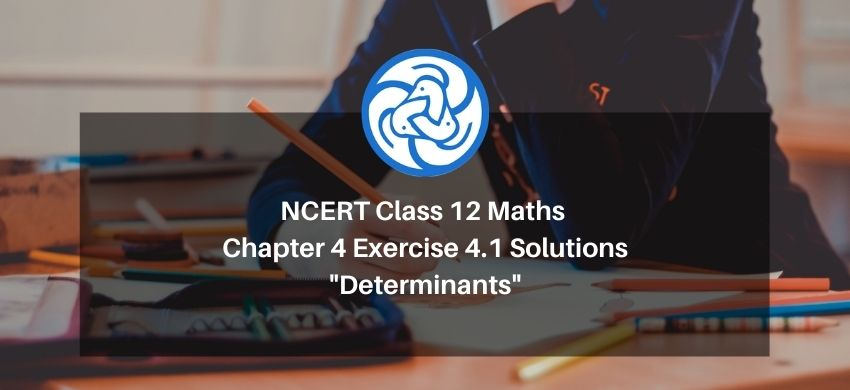Hey, are you a class 12 Student and Looking for Ways to Download Class 12 Maths Chapter 4 Exercise 4.1 Solutions? If Yes then you are at the right place.

All the solutions of Class 12 Math Chapter 4 exercise 4.1 is prepared by Kota’s top IITian’s Faculties by keeping Simplicity in mind.

If you want to score high in your class 12 Maths Exam then it is very important for you to have a good knowledge of all the important topics, so to learn and practice those topics you can use eSaral NCERT Solutions.

So, without wasting more time Let’s start.

### Download The PDF of NCERT Class 12 Math Chapter 4 Exercise 4.1 Solutions "Determinants"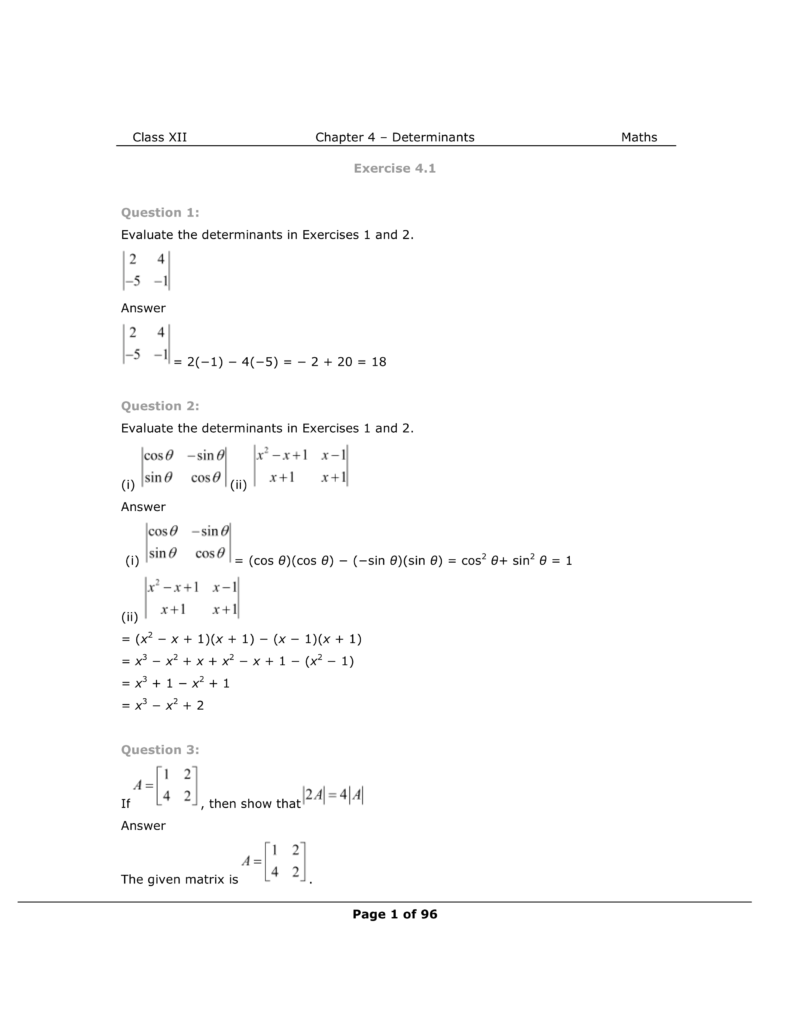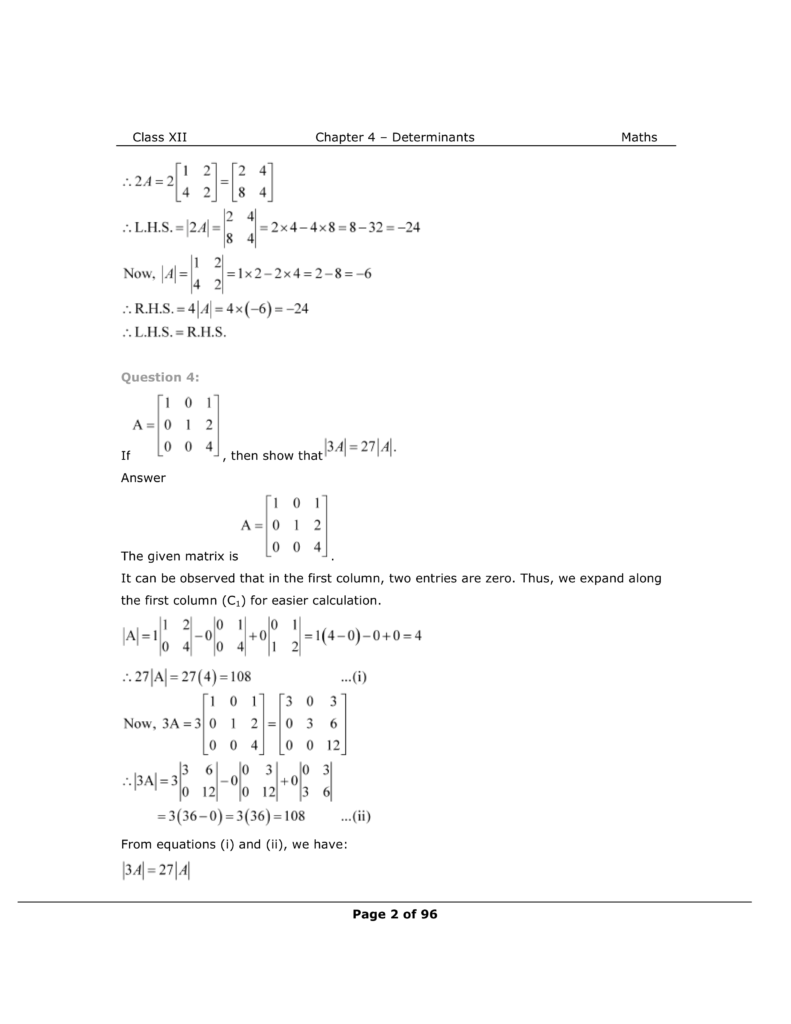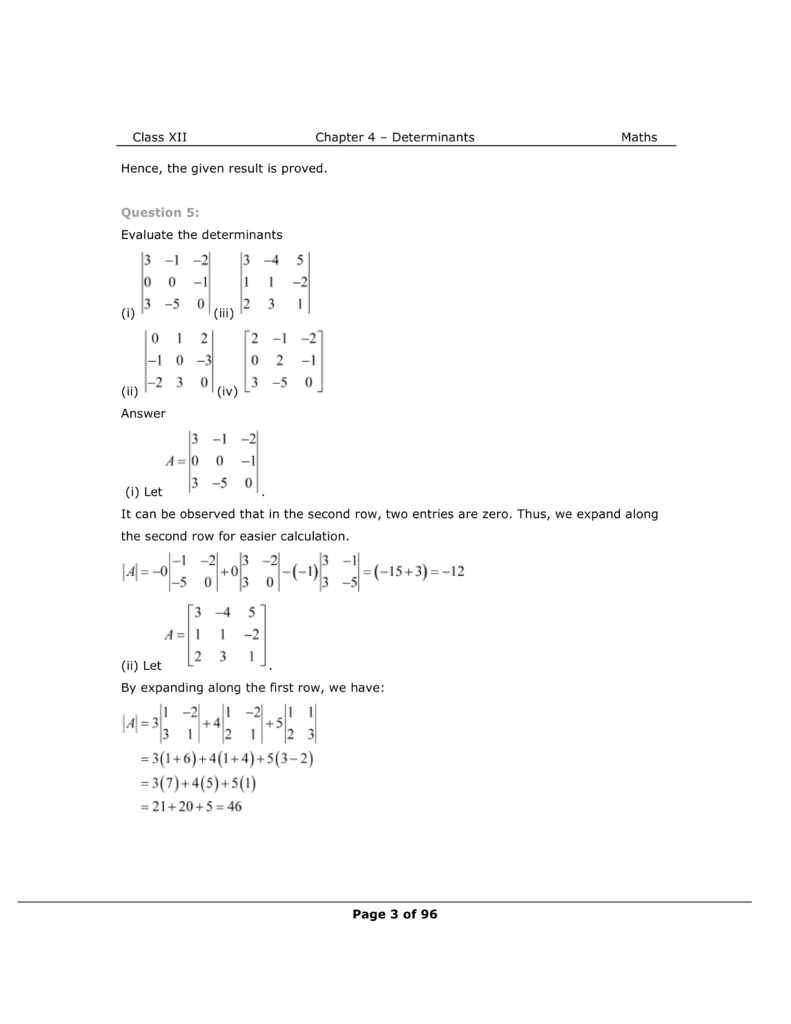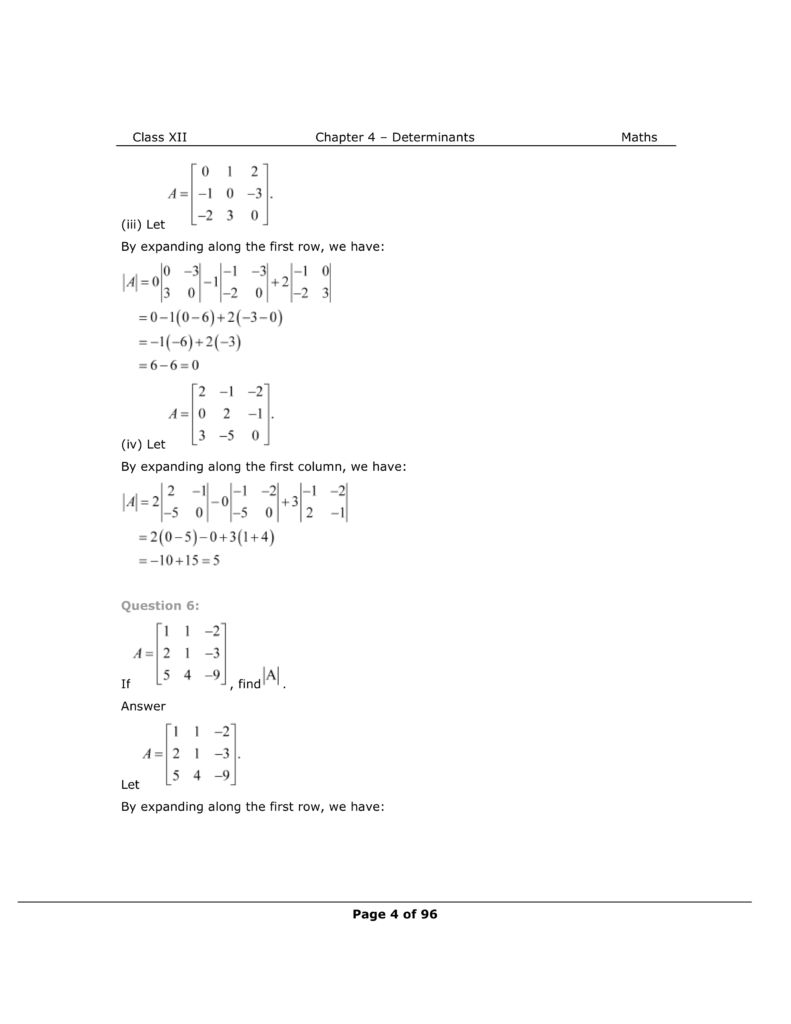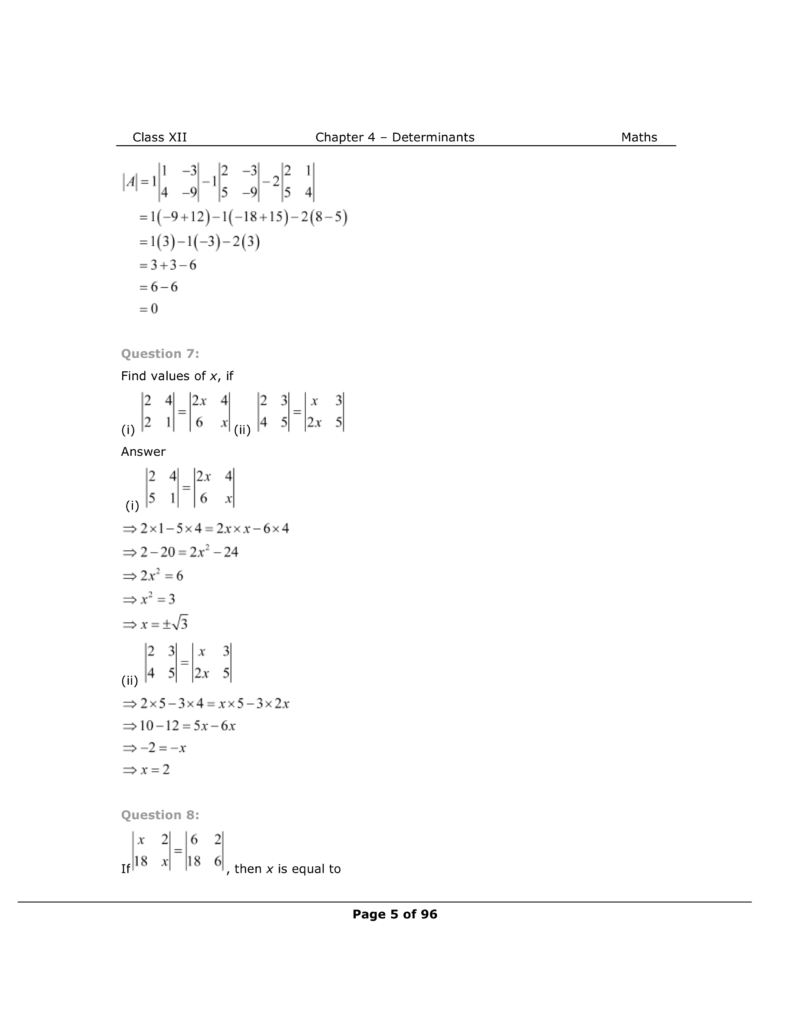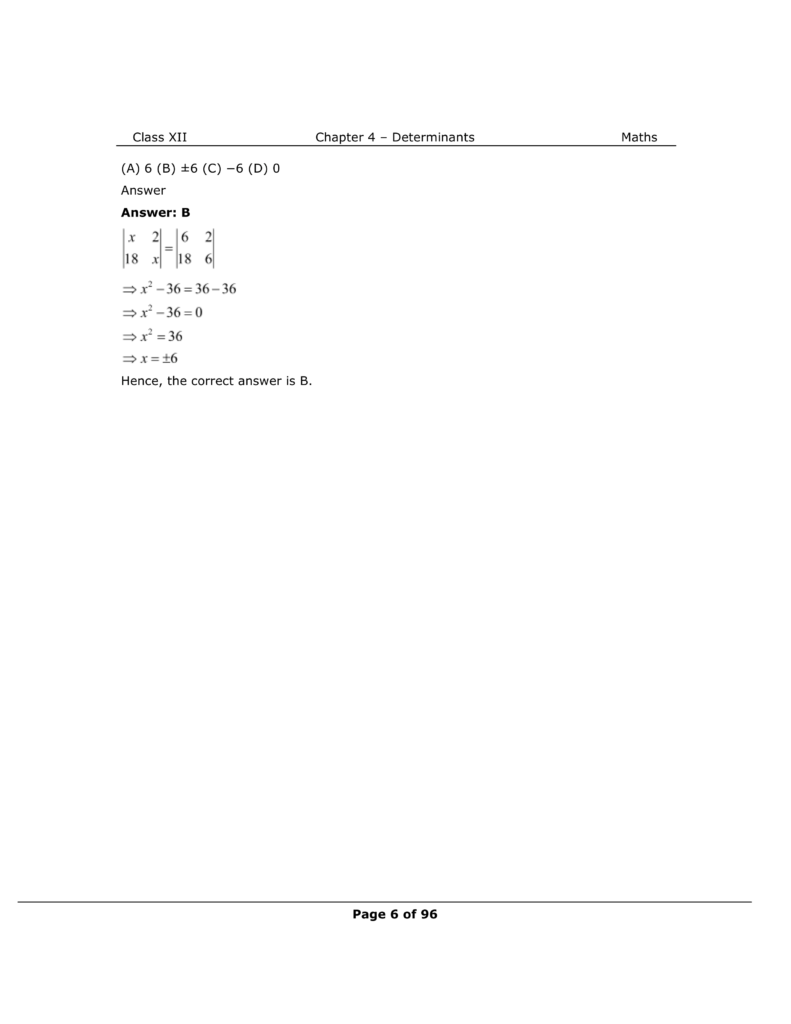NCERT Class 12 Math Book

NCERT Class 12 Maths Exemplar

Complete Solutions for Class 12 Maths chapter 4

Class 12 maths chapter 3 exercise 3.1 Solutions

Class 12 maths chapter 3 exercise 3.2 Solutions

Class 12 maths chapter 3 exercise 3.3 Solutions

Class 12 maths chapter 3 exercise 3.4 Solutions

Class 12 maths chapter 3 Miscellaneous exercise Solutions

If you have any Confusion related to Class 12 Maths Chapter 4 Exercise 4.1 Solutions then feel free to ask in the comments section down below.

To watch Free Learning Videos on Class 12 by Kota’s top Faculties Install the eSaral App